MCAT Physical : Optics

Example Questions

Example Question #1 : Velocity And Index Of Refraction

An incandescent light bulb is shown through a glass prism. The certain wavlength of the light is then directed into a glass cuvette containing an unknown concentration of protein. Commonly, this process is called spectroscopy and is used to determine the concentrations of DNA, RNA, and proteins in solutions. The indices of reflection of air, glass, and the solution are 1, 1.5, and 1.3, respectively.

The velocity of the light __________ when it moves from air to glass.

remains the same

cannot be determined

decreases

increases

decreases

Explanation:

This question asks us to consider the relationship between velocity and index of refraction of a medium. If we think back to the definition of index of refraction, we know that it is defined by the ratio of the velocity of light in a vacuum and the velocity of light in some other medium.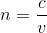n is the index of refraction, c is the speed of light in a vacuum, and v is the speed of light in the new medium.

We can see that n and v are inversely proportional, meaning that the higher the n, the lower the velocity. As the light moved from air (n =1) to glass (n = 1.5), the n increased, and thus the velocity must decrease because the speed of light in a vacuum is constant.

Example Question #1 : General Principles And Properties

An incandescent light bulb is shown through a glass prism. The certain wavlength of the light is then directed into a glass cuvette containing an unknown concentration of protein. Commonly, this process is called spectroscopy and is used to determine the concentrations of DNA, RNA, and proteins in solutions. The indices of reflection of air, glass, and the solution are 1, 1.5, and 1.3, respectively.

As light exits from the wall of the cuvette into the solution, its wavelength __________.

increases

decreases

remains the same

cannot be determined

increases

Explanation:

This question asks us to find the relationship between the wavelength and index of refraction. We will need to know two equations to compute the relationship between the two.

First, we need to relate velocity and index of refraction. The definition of index of refraction allows us to relate the two.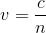We also know the relationship between velocity and wavelength.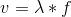We can now set these formulas equal to each other to find the relationship between wavelength and index of refraction.We can see that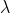and n are inversely related. If n decreases, the wavelength must increase. In our problem, light in moving from a higher index of refraction to a lower one, meaning the wavelength gets longer (increases).

Example Question #1 : Velocity And Index Of Refraction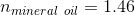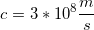How long will it take a photon to travel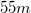through mineral oil?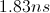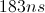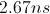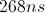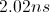Explanation:

The index of refraction is equal to the speed of light in a medium divided by the speed of light in a vacuum.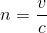We can find the time to travel a given distance by manipulating this equation and combining it with the equation for rate: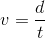.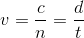Plug in the given values and solve for the velocity in the medium.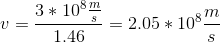Now we can return to the rate equation and solve for the time to travel.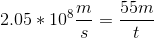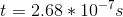We can recognize that the answer can be simplified by converting to nanoseconds.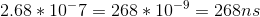Example Question #1 : Velocity And Index Of Refraction

The refractive index of medium A is 1.2, while that of medium B is 1.36. Through which medium does light travel faster and at what speed does it travel?

The speed of light is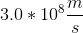.

Medium B at velocity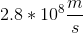The speed of light will bein either medium

Medium A at velocity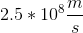Medium A at velocity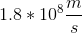Medium B at velocity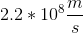Medium A at velocityExplanation:

The refractive index of a medium (n) is equal to the speed of light (c) divided by the velocity of light through the medium (v).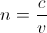Rearranging the equation allows us to see the relationship regarding v.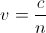The lower the refractive index, the faster the velocity of light. Medium A has the smaller refractive index. Light will travel faster through medium A at a velocity equal to the speed of light divided by the refractive index.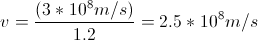Example Question #1 : Velocity And Index Of Refraction

Which of the following does not take place when a light wave travels from a medium with a high index of refraction into one with a lower index of refraction?

Wavelength increases

Velocity increases

The refracted light remains in phase with the incident wave

Frequency increases

The light ray bends away from the normal line

Frequency increases

Explanation:

As the wave travels into the less dense medium, it speeds up, bending away from the normal line. The index of refraction tells the ratio of the velocity in a vacuum in relation to the velocity the medium; thus, the velocity will be greater in a medium with a lower index of refraction.Frequency remains the same regardless of medium, however, since the velocity changes, the wavelength must accommodate this change.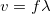If velocity increases and frequency remains constant, wavelength must also increase.

Finally, a phase shift only occurs when a light ray reflects from the surface of a more dense medium.

Example Question #201 : Mcat Physical Sciences

What is the index of refraction for a material in which light travels at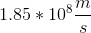?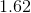Such a material does not exist, since the speed of light is constant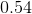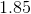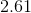Explanation:

Relevant equations: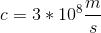To find index of refraction, divide the speed of light in a vacuum by the speed of light in the material: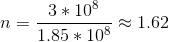Example Question #1 : Snell's Law

A monochromatic light ray passes from air (n = 1.00) into glass (n = 1.50) at an angle of 20o with respect to the normal. What is the approximate angle of refraction?

17o

24o

13o

27o

31o

13o

Explanation:

To compare angles of incidence and refraction, use Snell's law.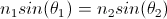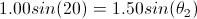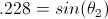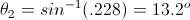Notice that as the light enters a more dense medium, it bends towards the normal.

Example Question #1 : Snell's Law

An incandescent light bulb is shown through a glass prism. The certain wavlength of the light is then directed into a glass cuvette containing an unknown concentration of protein. Commonly, this process is called spectroscopy and is used to determine the concentrations of DNA, RNA, and proteins in solutions. The indices of reflection of air, glass, and the solution are 1, 1.5, and 1.3, respectively.

The red light, selected for by the prism, is shown through air onto the glass cuvette at an angle of 30o to the normal. At what angle to normal does the light have when it is in the glass?

22o

19.5o

30o

45o

19.5o

Explanation:

This problem asks us to consider refraction, that is, the bending of light when it enters a new medium. Thinking back to our light formulas in physics, we know that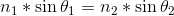, where n is the index of refraction of the medium and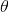is the angle the light ray makes to normal.

Using the information provided in the pre-question text and the question above, we can solve for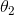.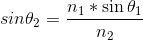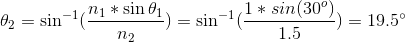Example Question #1 : Snell's Law

An incandescent light bulb is shown through a glass prism. The certain wavlength of the light is then directed into a glass cuvette containing an unknown concentration of protein. Commonly, this process is called spectroscopy and is used to determine the concentrations of DNA, RNA, and proteins in solutions. The indices of reflection of air, glass, and the solution are 1, 1.5, and 1.3, respectively.

Red light is selected for by the prism and shown onto the glass cuvette at an angle of 30o to the normal. At what angle to normal does the light travel when it is in the solution after it has moved through wall of the cuvette?

22.7o

45o

19.5o

35o

22.7o

Explanation:

Notice that, in this problem, there are two different changes in indices of refraction: air to glass and glass to solution; thus, we need to compute the angle change twice. First, let’s look at how the angle changes from air to glass. We know from Snell’s law that, where n is the index of refraction of the medium andis the angle the light ray makes to normal.

Rearranging, we can find the angle to normal in the glass.Now, we can use this angle and repeat the above equation to find the angle that the light enters the solution.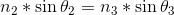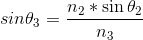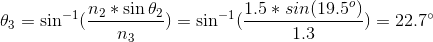Example Question #1 : Snell's Law

An incandescent light bulb is shown through a glass prism. The certain wavlength of the light is then directed into a glass cuvette containing an unknown concentration of protein. Commonly, this process is called spectroscopy and is used to determine the concentrations of DNA, RNA, and proteins in solutions. The indices of reflection of air, glass, and the solution are 1, 1.5, and 1.3, respectively.

If the solution had a higher protein concentration than expected, how would the angle of refraction change as light travels from the glass into the solution?

It would remain the same

It would be larger

It would be smaller

It would be larger or smaller, depending on the color of the light

It would be smaller

Explanation:

This question is asking us to consider what would happen if the solution were more concentrated, essentially, if there were more particles per unit volume (denser). If we think back to the definition of index of refraction, we know that it relates to the density of a medium. The denser the medium, the higher the index of refraction.Looking at Snell’s law, we can see the relationship between index of refraction and the angle of refraction.If the solution were more concentrated, n2 would increase, making the term on the right side of the equation smaller. The sin function of a smaller number gives a smaller angle; thus, as the concentration increases (and thus the index of refraction increases), the angle of refraction gets smaller.

All MCAT Physical Resources